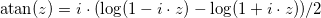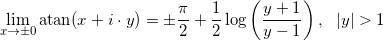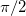# Branch Cuts and Signed Zeros in MATLAB

by on April 28, 2016

Signed zeros are important part of the IEEE754 standard for floating point arithmetic, commonly supported in hardware and in programming languages (C++, C, FORTRAN, etc.).

One of the areas where signed zeros play essential role is correct handling of branch cuts of special functions. In fact, it was one of the reasons why “negative zeros” were introduced in the first place.

The very unpleasant surprise is that MATLAB has poor support for this important feature.

I. Technically it allows user to create negative zeros:

>> x = -0 x = 0   >> 1/x ans = -Inf

The minus sign is not displayed in front of zero (well-known issue of MATLAB) but second operation shows that x is indeed negative.

II. (Real zeros) Sign of real zero is ignored in operations where it plays important role:

% MATLAB/Double precision >> atan(+0-1.5*1i) ans = 1.5707963267949 - 0.80471895621705i >> atan(-0-1.5*1i) ans = 1.5707963267949 - 0.80471895621705i
% MATLAB/Symbolic Math Toolbox (VPA) >> atan(vpa('+0-1.5*i')) ans = -1.570796327 - 0.8047189562 i >> atan(vpa('-0-1.5*i')) ans = -1.570796327 - 0.8047189562 i

In both cases sign of real zero is ignored, and the most amazingly is that different parts of MATLAB do not agree what sign zero has by default (VPA assumes zero is negative, the core function – zero is positive).

The atan has branch cut along the imaginary axis and sign of real part of argument is very important for result , so that correct answer should be:

% Advanpix toolbox - proper handling of branch cut: >> atan(mp(+0-1.5*1i)) ans = 1.570796326794896619231321691639751 - 0.8047189562170501873003796666130938i   >> atan(mp(-0-1.5*1i)) ans = -1.570796326794896619231321691639751 - 0.8047189562170501873003796666130938i

III. (Imaginary zeros) Complex numbers with zero imaginary part can be created in MATLAB only by using the complex command:

% Zero is ignored: >> z = -1-0i z = -1   >> isreal(z) ans = 1   % Complex with negative zero as imaginary part: >> z = complex(-1,-0) z = -1 + 0i >> 1/imag(z) ans = -Inf

Again minus sign is not displayed but zero is stored as negative. Let’s test it on branch cut of square root:

% MATLAB/Double precision >> sqrt(complex(-1,-0)) ans = 0 + 1i >> sqrt(complex(-1,+0)) ans = 0 + 1i
% MATLAB/Symbolic Math Toolbox (VPA) >> sqrt(vpa('-1+0*i')) ans = 1.0 i >> sqrt(vpa('-1-0*i')) ans = 1.0 i

Whereas correct results obviously are:

% Advanpix toolbox (correct result): >> sqrt(mp('-1-0i')) ans = 0 - 1i >> sqrt(mp('-1+0i')) ans = 0 + 1i

Unfortunately MATLAB ignores signed zeros in _all_ inverse trigonometric functions, sqrt, log and power (and apparently in special functions). This is super strange since it provides access to hardware floats and such basic functions have been implemented with correct handling of branch cuts for decades (e.g. as part of C standard).

***
Logarithmic form[5,6] ofgives expression for function values along branch cut:Sign of the real part of argument defines the sign ofin result.

### References

1. IEEE Computer Society (2008), IEEE Standard for Binary Floating-Point Arithmetic.
2. ISO/IEC 14882: Programming Language C++ (All revisions).
3. ISO/IEC 9899: Programming language C (All revisions).
4. JTC1/SC22/WG5: FORTRAN.
5. Kahan, W., “Branch Cuts for Complex Elementary Functions, or Much Ado About Nothing’s Sign Bit”, The State of the Art in Numerical Analysis, (Eds. Iserles and Powell), Clarendon Press, Oxford, 1987.
6. Mathematica. Inverse Trigonometric Functions.